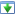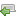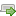Choose a version here. If you've not already started the tutorial, just go with the latest one.

 30 Aug 2014 Preview version Choose 5 Oct 2014 Explicitly adds a specific charset for htmlspecialchars(), and wraps it with a custom function Choose 16 Oct 2014 Updated redirect function to work with vhost subfolders Choose 4 Nov 2014 Minor improvements: added missing docblock, fixed security issue, CSS tweak. Switched hashing method to DEFAULT instead of BCRYPT, this is best practice. Choose 25 Nov 2014 Improve the notes on getting started, in particular choosing a programmer's editor. Added introduction to mod_rewrite rules. Choose 16 Aug 2018 Some bug fixes, remove compatibility library for earlier version of PHP Choose ♥
OK
NB: There are several versions of this tutorial, each successive one containing additional improvements. If you're in the middle of working through it, please check the versions panel above, to ensure you're not mixing code from different versions.

It is normal practice to link the main heading of a website to the home page, as this makes for a consistent navigation experience. So, let's do that now:`1`
` `
` `
`2`
`<h1>Blog title</h1>`
`<p>This paragraph summarises what the blog is about.</p>`
`1`
`2`
`3`
`4`
`<a href="index.php">`
` <h1>Blog title</h1>`
`</a>`
`<p>This paragraph summarises what the blog is about.</p>`

Next, let us add in some logic that interprets two carriage-returns in a post as a paragraph break. Here we go:`36`
`37`
`38`
` `
` `
` `
` `
`39`
`40`
`41`
`60`
`61`
`62`
`63`
` `
`64`
`65`
`66`
`// Let's get a row`
`\$row = \$stmt->fetch(PDO::FETCH_ASSOC);`
`?>`
`<!DOCTYPE html>`
`<html>`
` <?php echo \$row['created_at'] ?>`
` </div>`
` <p>`
` <?php echo htmlEscape(\$row['body']) ?>`
` </p>`
` </body>`
`</html>`
`36`
`37`
`38`
`39`
`40`
`41`
`42`
`43`
`44`
`45`
`60`
`61`
`62`
`63`
`64`
`65`
`66`
`67`
`// Let's get a row`
`\$row = \$stmt->fetch(PDO::FETCH_ASSOC);`
`// Swap carriage returns for paragraph breaks`
`\$bodyText = htmlEscape(\$row['body']);`
`\$paraText = str_replace("\n", "</p><p>", \$bodyText);`
`?>`
`<!DOCTYPE html>`
`<html>`
` <?php echo \$row['created_at'] ?>`
` </div>`
` <p>`
` <?php // This is already escaped, so doesn't need further escaping ?>`
` <?php echo \$paraText ?>`
` </p>`
` </body>`
`</html>`

Now, the default date style used around the application isn't very readable, so let's improve that now:`31`
`32`
`33`
`34`
`35`
`36`
`37`
` <?php echo htmlEscape(\$row['title']) ?>`
` </h2>`
` <div>`
` <?php echo \$row['created_at'] ?>`
` </div>`
` <p>`
` <?php echo htmlEscape(\$row['body']) ?>`
`31`
`32`
`33`
`34`
`35`
`36`
`37`
` <?php echo htmlEscape(\$row['title']) ?>`
` </h2>`
` <div>`
` <?php echo convertSqlDate(\$row['created_at']) ?>`
` </div>`
` <p>`
` <?php echo htmlEscape(\$row['body']) ?>`
`50`
`51`
`52`
`{`
` return htmlspecialchars(\$html, ENT_HTML5, 'UTF-8');`
`}`
`50`
`51`
`52`
`53`
`54`
`55`
`56`
`57`
`58`
`59`
`60`
`{`
` return htmlspecialchars(\$html, ENT_HTML5, 'UTF-8');`
`}`
`function convertSqlDate(\$sqlDate)`
`{`
` /* @var \$date DateTime */`
` \$date = DateTime::createFromFormat('Y-m-d', \$sqlDate);`
` return \$date->format('d M Y');`
`}`
`57`
`58`
`59`
`60`
`61`
`62`
`63`
` <?php echo htmlEscape(\$row['title']) ?>`
` </h2>`
` <div>`
` <?php echo \$row['created_at'] ?>`
` </div>`
` <p>`
` <?php // This is already escaped, so doesn't need further escaping ?>`
`57`
`58`
`59`
`60`
`61`
`62`
`63`
` <?php echo htmlEscape(\$row['title']) ?>`
` </h2>`
` <div>`
` <?php echo convertSqlDate(\$row['created_at']) ?>`
` </div>`
` <p>`
` <?php // This is already escaped, so doesn't need further escaping ?>`

Now we've done a bit of tidying, let's tackle a bigger item of functionality. We need to allow users to comment on articles, so let's make the necessary database changes to prepare for that:`54`
`55`
`56`
` `
` `
` `
` `
` `
` `
` `
` `
` `
` `
` `
` `
` `
` `
` `
` `
` `
` `
` `
` `
` `
` `
` `
` `
` `
` `
` `
` `
` `
` `
` `
` `
` `
` `
` `
` `
` `
` `
` `
`57`
` 1,`
` date('now', '-13 days')`
` )`
`;`
`54`
`55`
`56`
`57`
`58`
`59`
`60`
`61`
`62`
`63`
`64`
`65`
`66`
`67`
`68`
`69`
`70`
`71`
`72`
`73`
`74`
`75`
`76`
`77`
`78`
`79`
`80`
`81`
`82`
`83`
`84`
`85`
`86`
`87`
`88`
`89`
`90`
`91`
`92`
`93`
`94`
`95`
`96`
` 1,`
` date('now', '-13 days')`
` )`
`;`
`DROP TABLE IF EXISTS comment;`
`CREATE TABLE comment (`
` id INTEGER PRIMARY KEY AUTOINCREMENT NOT NULL,`
` post_id INTEGER NOT NULL,`
` created_at VARCHAR NOT NULL,`
` name VARCHAR NOT NULL,`
` website VARCHAR,`
` text VARCHAR NOT NULL`
`);`
`INSERT INTO`
` comment`
` (`
` post_id, created_at, name, website, text`
` )`
` VALUES(`
` 1,`
` date('now', '-10 days'),`
` 'Jimmy',`
` 'http://example.com/',`
` "This is Jimmy's contribution"`
` )`
`;`
`INSERT INTO`
` comment`
` (`
` post_id, created_at, name, website, text`
` )`
` VALUES(`
` 1,`
` date('now', '-8 days'),`
` 'Jonny',`
` 'http://anotherexample.com/',`
` "This is a comment from Jonny"`
` )`
`;`
`49`
`50`
`51`
`52`
`53`
` `
`54`
`55`
`56`
`57`
`58`
`59`
` `
` `
` `
` `
` `
` `
`60`
`61`
`62`
`93`
`94`
`95`
`96`
`97`
`98`
` `
` `
` `
` `
` `
` `
` `
`99`
`100`
`101`
`}`
`// See how many rows we created, if any`
`\$count = null;`
`if (!\$error)`
`{`
` \$sql = "SELECT COUNT(*) AS c FROM post";`
` \$stmt = \$pdo->query(\$sql);`
` if (\$stmt)`
` {`
` \$count = \$stmt->fetchColumn();`
` }`
`}`
` <?php else: ?>`
` <div class="success box">`
` The database and demo data was created OK.`
` <?php if (\$count): ?>`
` <?php echo \$count ?> new rows were created.`
` <?php endif ?>`
` </div>`
` <?php endif ?>`
` </body>`
`49`
`50`
`51`
`52`
`53`
`54`
`55`
`56`
` `
` `
`57`
`58`
`59`
`60`
`61`
`62`
`63`
`64`
`65`
`66`
`67`
`93`
`94`
`95`
`96`
`97`
`98`
`99`
`100`
`101`
`102`
`103`
`104`
`105`
`106`
`107`
`108`
`}`
`// See how many rows we created, if any`
`\$count = array();`
`foreach(array('post', 'comment') as \$tableName)`
`{`
` if (!\$error)`
` {`
` \$sql = "SELECT COUNT(*) AS c FROM " . \$tableName;`
` \$stmt = \$pdo->query(\$sql);`
` if (\$stmt)`
` {`
` // We store each count in an associative array`
` \$count[\$tableName] = \$stmt->fetchColumn();`
` }`
` }`
`}`
` <?php else: ?>`
` <div class="success box">`
` The database and demo data was created OK.`
` <?php foreach (array('post', 'comment') as \$tableName): ?>`
` <?php if (isset(\$count[\$tableName])): ?>`
` <?php // Prints the count ?>`
` <?php echo \$count[\$tableName] ?> new`
` <?php // Prints the name of the thing ?>`
` <?php echo \$tableName ?>s`
` were created.`
` <?php endif ?>`
` <?php endforeach ?>`
` </div>`
` <?php endif ?>`
` </body>`

Since this involves database changes, we'll need to delete our database file, and re-run the installer. So, delete the file in data/data.sqlite and then reinstall by visiting `http://localhost/install.php` again.

You might ask why we are putting so much effort into an installer when we're nowhere near having a piece of finished software. The answer is that this installer is for us, the developers, and not for end users. Thus, it is something we want to set up reasonably early in our development lifecycle; whenever a database change is made, we want to be able to recreate our useful test data quickly and easily.

Now we're ready to start adding some comment-related changes. To start with, let's add a count of comments on the front page. This uses the SQL command `COUNT()` to count the number of rows that would be returned by a query:`32`
`33`
`34`
` `
` `
`35`
`36`
`37`
` </h2>`
` <div>`
` <?php echo convertSqlDate(\$row['created_at']) ?>`
` </div>`
` <p>`
` <?php echo htmlEscape(\$row['body']) ?>`
`32`
`33`
`34`
`35`
`36`
`37`
`38`
`39`
` </h2>`
` <div>`
` <?php echo convertSqlDate(\$row['created_at']) ?>`
` (<?php echo countCommentsForPost(\$row['id']) ?> comments)`
` </div>`
` <p>`
` <?php echo htmlEscape(\$row['body']) ?>`
`58`
`59`
`60`
` return \$date->format('d M Y');`
`}`
`58`
`59`
`60`
`61`
`62`
`63`
`64`
`65`
`66`
`67`
`68`
`69`
`70`
`71`
`72`
`73`
`74`
`75`
`76`
`77`
`78`
`79`
`80`
`81`
`82`
`83`
`84`
`85`
` return \$date->format('d M Y');`
`}`
`/**`
` * Returns the number of comments for the specified post`
` *`
` * @param integer \$postId`
` * @return integer`
` */`
`function countCommentsForPost(\$postId)`
`{`
` \$pdo = getPDO();`
` \$sql = "`
` SELECT`
` COUNT(*) c`
` FROM`
` comment`
` WHERE`
` post_id = :post_id`
` ";`
` \$stmt = \$pdo->prepare(\$sql);`
` \$stmt->execute(`
` array('post_id' => \$postId, )`
` );`
` return (int) \$stmt->fetchColumn();`
`}`

Also, we'll add a comment listing to individual post pages:`83`
`84`
`85`
` return (int) \$stmt->fetchColumn();`
`}`
`83`
`84`
`85`
`86`
`87`
`88`
`89`
`90`
`91`
`92`
`93`
`94`
`95`
`96`
`97`
`98`
`99`
`100`
`101`
`102`
`103`
`104`
`105`
`106`
`107`
`108`
`109`
` return (int) \$stmt->fetchColumn();`
`}`
`/**`
` * Returns all the comments for the specified post`
` *`
` * @param integer \$postId`
` */`
`function getCommentsForPost(\$postId)`
`{`
` \$pdo = getPDO();`
` \$sql = "`
` SELECT`
` id, name, text, created_at, website`
` FROM`
` comment`
` WHERE`
` post_id = :post_id`
` ";`
` \$stmt = \$pdo->prepare(\$sql);`
` \$stmt->execute(`
` array('post_id' => \$postId, )`
` );`
` return \$stmt->fetchAll(PDO::FETCH_ASSOC);`
`}`
`63`
`64`
`65`
` `
` `
` `
` `
` `
` `
` `
` `
` `
` `
` `
` `
` `
` `
` `
` `
` `
` `
`66`
`67`
` <?php // This is already escaped, so doesn't need further escaping ?>`
` <?php echo \$paraText ?>`
` </p>`
` </body>`
`</html>`
`63`
`64`
`65`
`66`
`67`
`68`
`69`
`70`
`71`
`72`
`73`
`74`
`75`
`76`
`77`
`78`
`79`
`80`
`81`
`82`
`83`
`84`
`85`
` <?php // This is already escaped, so doesn't need further escaping ?>`
` <?php echo \$paraText ?>`
` </p>`
` <h3><?php echo countCommentsForPost(\$postId) ?> comments</h3>`
` <?php foreach (getCommentsForPost(\$postId) as \$comment): ?>`
` <?php // For now, we'll use a horizontal rule-off to split it up a bit ?>`
` <hr />`
` <div class="comment">`
` <div class="comment-meta">`
` Comment from`
` <?php echo htmlEscape(\$comment['name']) ?>`
` on`
` <?php echo convertSqlDate(\$comment['created_at']) ?>`
` </div>`
` <div class="comment-body">`
` <?php echo htmlEscape(\$comment['text']) ?>`
` </div>`
` </div>`
` <?php endforeach ?>`
` </body>`
`</html>`

So, give that a whirl. You will want to check the new count on the homepage, and the comments feature on individual posts. How's that looking?